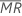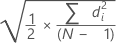# Methods for Between/Within Capability Sixpack

## Estimating standard deviation

Between/within capability analysis is based on the following four standard deviations:

### Within-subgroup standard deviation

σwithin is an estimate of the variation within subgroups (for example, one shift, one operator, or one material batch). Minitab estimates σwithin using one of the following methods:

• Pooled standard deviation:

where:

###### Note

If you change the default method and choose not to use the unbiasing constant, σwithin is estimated by Sp.

TermDescription
dDegrees of freedom for Sp= Σ (ni- 1)
Xij jth observation in the ith subgroup
iMean of the ith subgroup
niNumber of observations in the ith subgroup
C4(d+1)Unbiasing constant
Γ(·)Gamma function
• Average of subgroup ranges (Rbar):

where:

If n are all the same:

TermDescription
riRange of the ith subgroup
d2 (ni) An unbiasing constant read from a table (for more information, see the section Unbiasing constants d2(), d3(), and d4()
d3 (ni) An unbiasing constant read from a table (for more information, see the section Unbiasing constants d2(), d3(), and d4()
niNumber of observations in the ith subgroup
• Average of subgroup standard deviations (Sbar):

where:

###### Note

If you change the default setting and do not use the unbiasing constant, σwithin is estimated by Σ Si / number of subgroups.

TermDescription
C4(ni)Unbiasing constant (as defined for pooled standard deviation)
SiStandard deviation of subgroup i
niNumber of observations in the ith subgroup

### Between-subgroup standard deviation

σBetween is an estimate of the variation between subgroups (for example, subgroups collected at set intervals, batches, or by different operators).

σ2Xbar is estimated using one of the following methods:
• Average of moving range:

where:

TermDescription
RiThe ith moving range
wThe number of observations used in the moving range. The default is w = 2.
d2(w)An unbiasing constant read from a table (for more information, see the section Unbiasing constants d2(), d3(), and d4()
• Median of moving range:

where:

TermDescription
MRiThe ith moving rangeMedian of the MRi
wThe number of observations used in the moving range. The default is w = 2.
d4(w)An unbiasing constant read from a table (for more information, see the section Unbiasing constants d2(), d3(), and d4()
• Square root of mean squared successive differences (MSSD):
###### Note

If you change the default setting and do not use the unbiasing constant, σwithin is estimated byTermDescription
diDifferences of successive group means
C4(ni)Unbiasing constant (as defined for the pooled standard deviation)
C4'(ni)Unbiasing constant ≈ c4(ni). For more information, see the section Unbiasing constant c4'().
NTotal number of observations
niNumber of observations in the ith subgroup

### Between/within standard deviation

TermDescription
σ2BetweenVariance between subgroups
σ2withinVariance within subgroups

### Overall standard deviation

where:

###### Note

By default, Minitab does not use the unbiasing constant when estimating σoverall. σoverall is estimated by S. If you want to estimate overall standard deviation using the unbiasing constant, you can change this option on the Estimate subdialog box when you perform the capability analysis. If you always want Minitab to use the unbiasing constant by default, choose File > Options > Control Charts and Quality Tools > Estimating Standard Deviation and select the appropriate options.

TermDescription
XijThe jth observation in the ith subgroup
Process mean
niNumber of observations in the ith subgroup
C4 (N)Unbiasing constant (as defined for the pooled standard deviation)
N (or Σ ni)Total number of observations

## Box-Cox transformation

The Box-Cox transformation estimates a lambda value, as shown in the following table, which minimizes the standard deviation of a standardized transformed variable. The resulting transformation is Yλ when λ ҂  0 and ln Y when λ = 0.

The Box-Cox method searches through many types of transformations. The following table shows some common transformations where Y' is the transform of the data Y.

Lambda (λ) value Transformation
By using this site you agree to the use of cookies for analytics and personalized content.  Read our policy